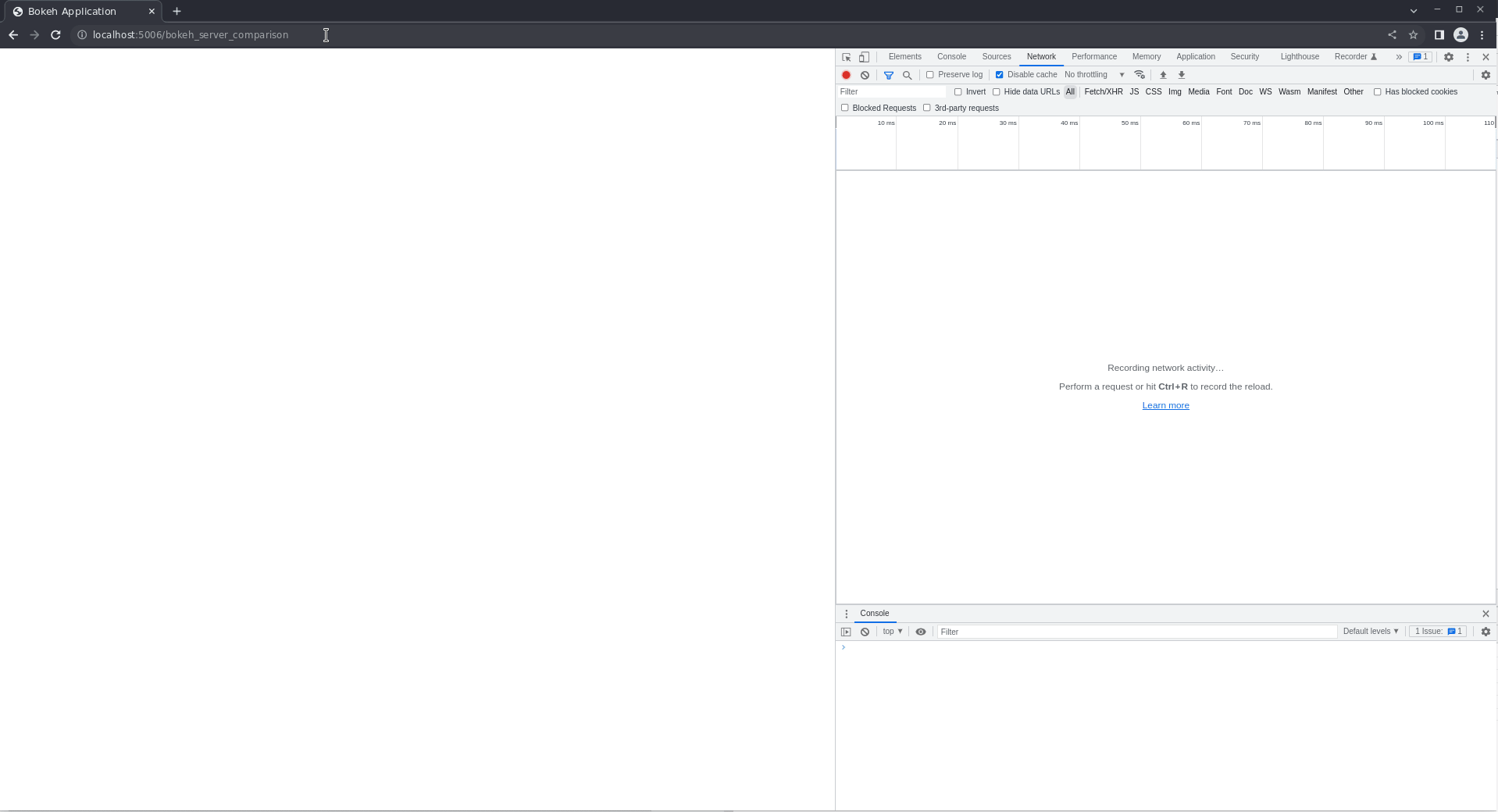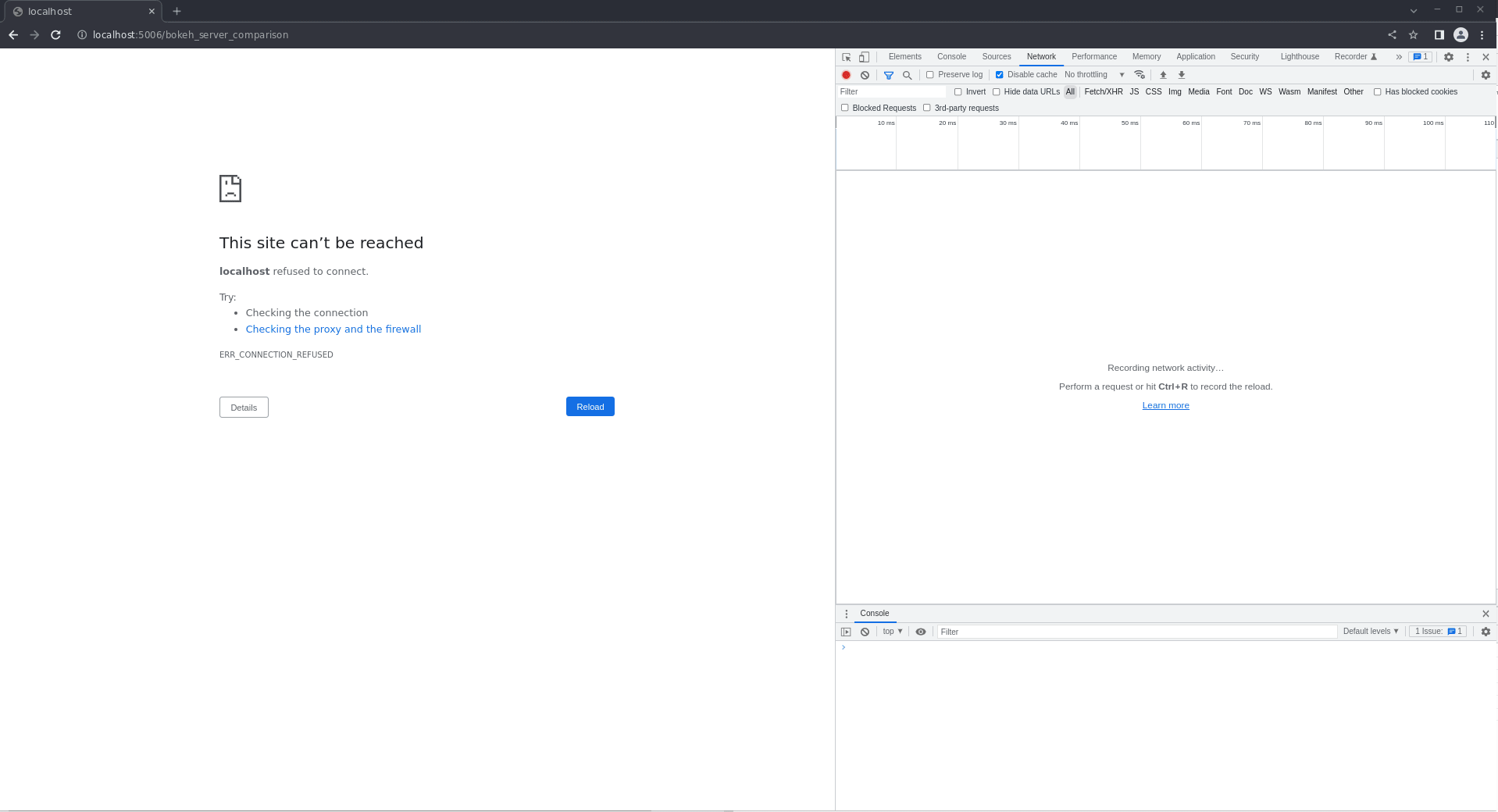# Performance comparison between v2 and v3

Hi, This is my first post, so thanks for the great work.

My team has been using bokeh v2 + Panel for 3 years to create very useful dashboards. However, serious performance issues appear when many layout elements (column, row, tabs, …) are nested (as mentioned in the documentation).

Excited by the announcements about the great performance improvement of the Bokeh 3 layout system, I decided to make some comparisons with v2. The file creates nested tabs containing a 2x2 grid of scatter plots made of row and column elements.

Unfortunately, the performance I got was worse in v3 than in v2.. Here are the results I got:

V2:V3:
See first reply, because I can attach only one media file.

I assume that I’m doing something wrong, but I don’t know where is the problem. The following script is used to test both versions. Only Tabs are imported differently regarding bokeh version.

``````# main.py

import numpy as np
import pandas as pd

from bokeh.plotting import curdoc, figure
from bokeh.layouts import column, row
from bokeh.models import Tabs, Band, ColumnDataSource, Div
from bokeh.util import version

BOKEH_VERSION = int(version.base_version())

if BOKEH_VERSION >= 3:
from bokeh.models import TabPanel as Panel
else:
from bokeh.models import Panel

def bk_version():
return Div(
text=f"""
<h1>Bokeh { BOKEH_VERSION }</h1>
"""
)

def scatter_plot():
N = 200
x = np.random.random(size=N) * 100
y = np.random.random(size=N) * 100
colors = np.array(
[[r, g, 150] for r, g in zip(50 + 2 * x, 30 + 2 * y)], dtype="uint8"
)

TOOLS = "hover,crosshair,pan,wheel_zoom,zoom_in,zoom_out,box_zoom,undo,redo,reset,tap,save,box_select,poly_select,lasso_select,"

p = figure(tools=TOOLS)
return p

def create_panel(id=None, ncols=2, plots_per_col=3):
return Panel(
child=create_grid(ncols=ncols, nrows=plots_per_col),
title=f"Tab {id or np.random.rand()}",
)

def create_grid(ncols=2, nrows=2):
return row(*[column(*[scatter_plot() for k in range(nrows)]) for k in range(ncols)])

def create_tabs(n, parent=None, ncols=2, plots_per_col=3):
return Tabs(
tabs=[
create_panel(id=f"{parent}.{i}", ncols=ncols, plots_per_col=plots_per_col)
for i in range(n)
]
)

def create_parent_tabs(n, subtabs=2, ncols=2, plots_per_col=3):
return Tabs(
tabs=[
Panel(
child=create_tabs(
subtabs, parent=i, ncols=ncols, plots_per_col=plots_per_col
),
title=f"SuperTab {i}",
)
for i in range(n)
]
)

if __name__.startswith("bokeh_app_"):

tabs = create_parent_tabs(
4,
subtabs=3,
ncols=2,
plots_per_col=2,
)

``````

The tests were performed using python 3.11 and bokeh server from two virtual environments using the following requirements files:

``````# requirements_v2.txt
bokeh==2.4.3
pandas>=1.5.2
``````
``````# requirements_v3.txt
bokeh==3.0.2
pandas>=1.5.2
``````

Both servers were launched from independent virtual environments with the following command:

``````\$ bokeh serve ./ --allow-websocket-origin="*:5006"
``````

Any ideas on what might be causing this? Is the way I am using bokeh server wrong?

If I missed something necessary to reproduce the issue, please let me know.